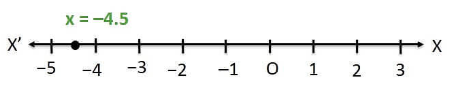Newbie

# Give the geometric representations of 2x+9 = 0 as an equation (i) in one variable. Q.2(1)

• 0

Sir please help me to solve the ncert class 9th solution of chapter linear equations in two variables .How I solve this question of exercise 4.4 question number 2(1). Find the simplest and easiest solution of this question. also find the best solution of this question.Give the geometric representations of 2x+9 = 0 as an equation (i) in one variable

Share

1. (i) In one variable,

2x+9 = 0

2x = -9

x = -9/2

x = -4.5• 0